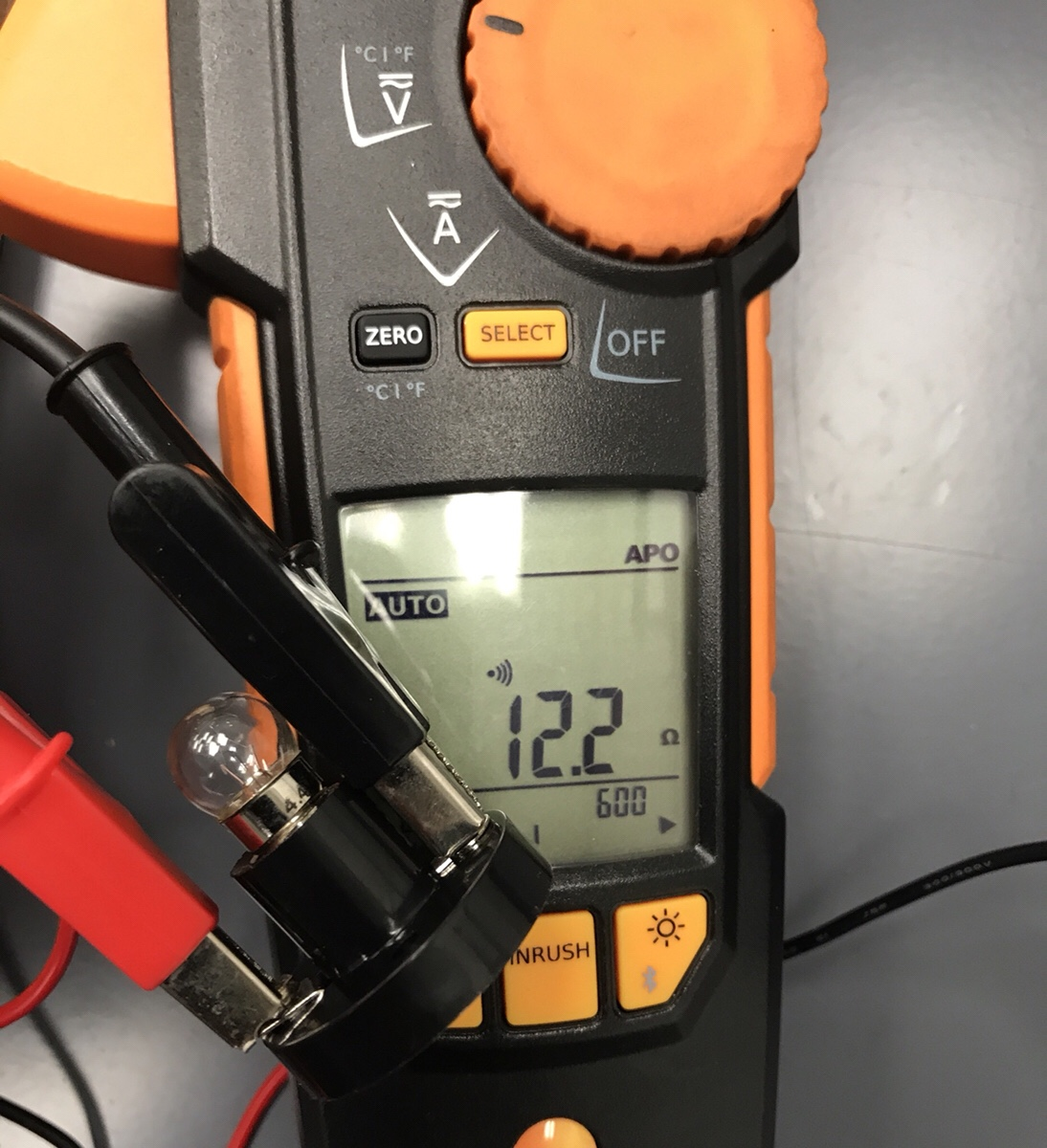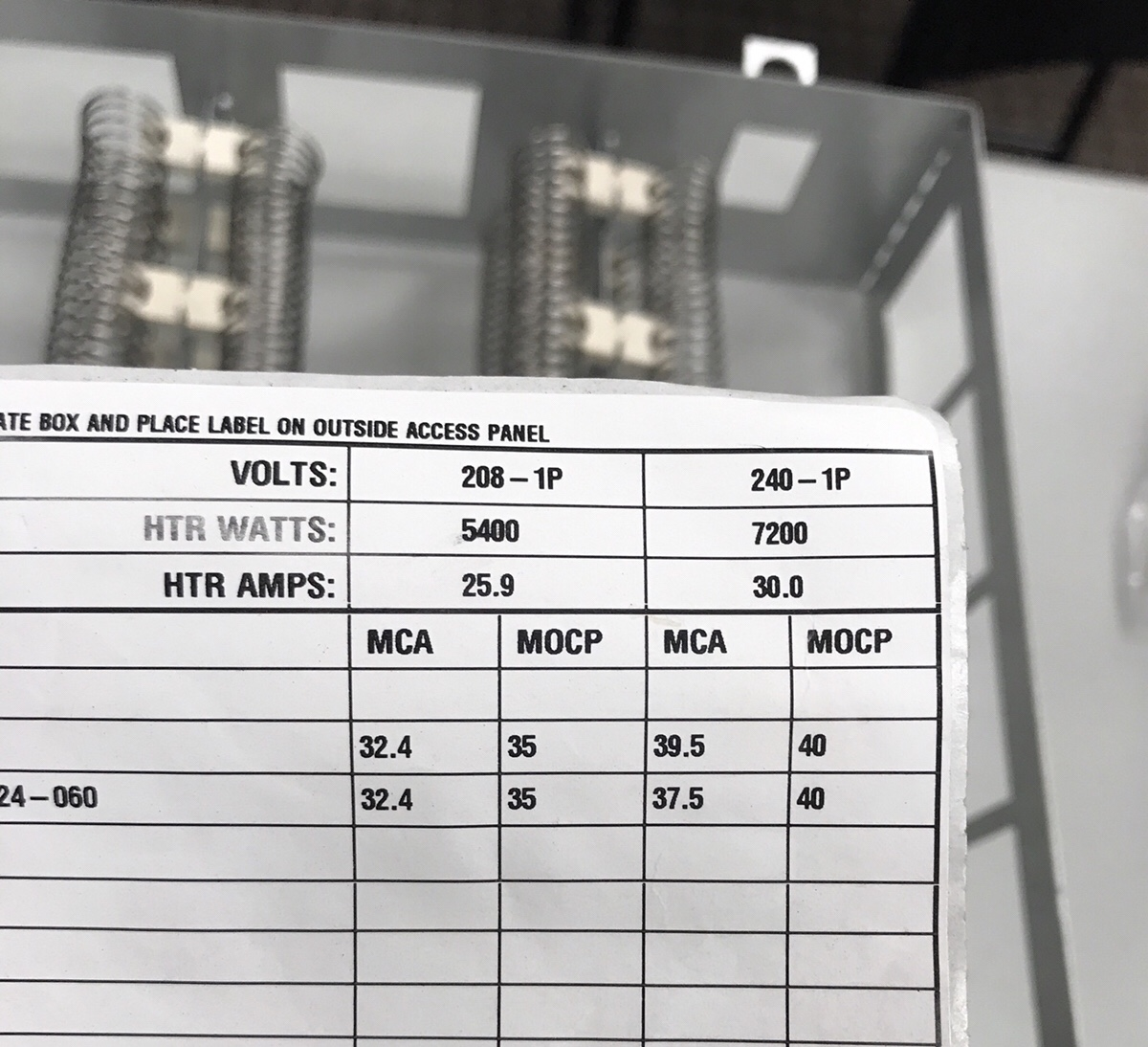## Mistakes in Measuring OhmsIn HVAC and electrical school, one of the first things you learn about electricity is Ohm’s law:

Volts = Amps x OhmsPretty simple Right? and Watt’s law is just as easy:

Watts = Volts x Amps

With this new found knowledge the student walks confidently into the real world with two equations and some elementary Algebra skills expecting to be able to predict Volts, Amps, Watts and Ohms using this secret knowledge.

Then they Ohm out a residential compressor or other single phase motor windings for the first time…

They might read something like:

Start to Common = 4.2 Ohms

Run to Common = 2.6 Ohms

Start to Run = 6.9 Ohms

So they measure their voltage, grab a calculator and calculate.

Run Winding 243V ÷ 2.6Ω = 93.4A

Start Winding 243V ÷ 4.2Ω = 57.85A

Well, THAT makes no sense…. so they stop using Ohms law and settle with believing that electricity is magic and ohms law is broken.

In actuality, Ohms law does work it’s just that the loads we measure vary and don’t all behave in the same way.

In the case above, a compressor/fan motor/contactor coil etc… is an inductive load. That means that its job is to convert electrical energy to electromagnetic force. While an inductive load does have SOME resistance when de-energized that can be measured with an Ohmmeter, the majority of the electrical resistance only shows up once current is applied.

In an inductive (magnetic) load, this resistance that shows up once it’s powered on is called inductive reactance and is still measured in Ohms.

In the case of the example above, the run winding is connected directly between L1 & L2 and when the circuit is completed by the contactor in the example above the run winding WILL actually draw really high amps for a split second (first electrical cycle) until the inductive reactance kicks in.

In the start winding the current is limited by a combination of resistance, inductive reactance and the capacitance of the capacitor. Add in the fact that the applied Voltage across the run winding is actually higher than the L1 – L2 voltage due to back EMF (CEMF) and its enough to confuse anyone.

Then you throw in Power Factor to the mix in an inductive load… this means that even when we multiply Volts x Amps in an inductive AC circuit what we see in VA isn’t necessarily what you get in Watts.

It takes a lot of measurements and math to figure this all out and unless you are an engineer it’s much easier to measure what you have on a functioning component rather than attempting to calculate amperage and wattage based on voltage and ohms.

However…

We are taught that resistive loads (loads that create light and heat) are much more simple. There is none of this inductive reactance or power factor nonsense in a resistive load like a light bulb or a heat strip.

But wait… there’s moreSo this light bulb is measuring 12.2 Ohms confirmed with two different meters and it is rated at 14.4 volts. So we do the simple math:

Amps = 14.4v ÷ 12.2Ω

Therefore Amps = 1.18
So we put it to the test by feeding this bulb exactly 14.4V from a DC power supplyAnnnnddd… Not even close

We expected an amperage of 1.18 and got an amperage of 0.1

SO WHAT THE HECK IS GOING ON?

Incandescent light bulbs are a resistive load but they are also made with a filament of the element tungsten which has PTCR (positive temperature coefficient resistor) properties. This means that the resistance of a tungsten incandescent light bulb goes up by 10 to 15 times from its cold temperature to its hot temperature. In the case of the bulb above we now its cold resistance is 12.2 ohms, but by working ohms law backward we can also tell that its HOT resistance is 142.57 ohms which means that the hot resistance is 11.6 times higher than cold in this particular instance.Not all resistive loads behave in this way though. Let’s look at a heat strip.Amps = 240v ÷ 15.5Ω

Amps = 15.48

Watts = 240v x 15.48

Watts = 3715 based on Ohms & Watts law

Don’t worry… This is a 7.2kw (7200 Watt) heat strip divided into two 3600 watt strips and we are only reading one half (3.6kw)

To bench test it further I applied one-tenth of the designed voltage (24 Volts) to see how it would respond.1.532a @ 24v = 15.32a @ 240v

The reason the math still doesn’t line up perfectly is that even in a heat strip the resistance increases as the temperature increases but in a much smaller fashion than in a light bulb.

When cold the math predicts it is a 3.7kw heater, when warm at 24v applied it predicts 3.67kw and at 240v the resistance increases to its full rating at 3.6kw.

All of this to say that Ohms & Watts law are useful and accurate but they are impacted by real “under load” forces in such a way that it much more realistic to make measurements on a functioning device and work backward than to use ohms to work upwards from an ohm bench test and a voltage.

— Bryan

This site uses Akismet to reduce spam. Learn how your comment data is processed.

Scroll to top
Translate »

### Daily Tech Tip

Get the (near) daily Tech Tip email right in your inbox!